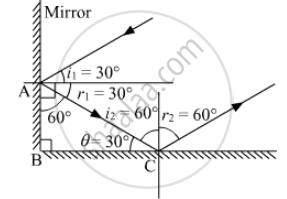SSC (English Medium) Class 8Maharashtra State Board
Share

# Draw a Figure Describing the Following. - SSC (English Medium) Class 8 - Science

ConceptConcept of Reflection of Light

#### Question

Draw a figure describing the following.

The reflecting surface of two mirrors make an angle of 90 with each other. If a ray incident of one mirror has an angle of incidents of 30, draw the ray reflected from the second mirror. What will be its angle of reflection?

#### Solution

Let r2 be the angle of reflection from the second mirror.From laws of reflection,

angle i _1 = angle r_1 = 30°

Now in triangle ABC

θ = 180° - 90° - 60° = 30°

⇒ i2 = 60°

Hence , r2 = 60°

The angle of reflection of incident ray is 60.

Is there an error in this question or solution?

#### APPEARS IN

Balbharati Solution for Balbharati Class 8 Science - General Science (2018 to Current)
Chapter 16: Reflection of Light
Exercise | Q: 2 | Page no. 115
Solution Draw a Figure Describing the Following. Concept: Concept of Reflection of Light.
S